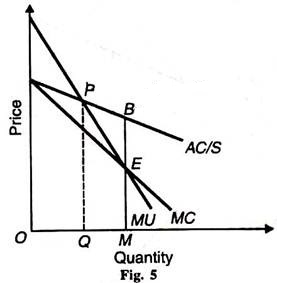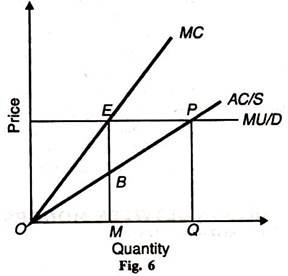# Difference between Monopsony and Perfect Competition

(c) Figure 5 illustrates the case of a decreasing supply price where the average and marginal cost curves slope downward. Here the monopolist buys more than under perfect competition at a lower price. The monopolist equilibrium is at E. MB is the monopsony price which is less than the perfectly competitive price PQ, but the monopolistic amount OM is higher than the perfectly competitive amount OQ.(d) If the demand curve is perfectly elastic as in Figure 6 the monopsony price will be exactly half the competitive price, MB= ½QP. The monopolist will also buy exactly half the perfectly competitive output OM=½OQ.